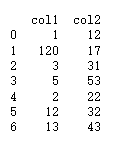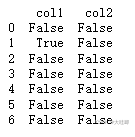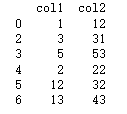• 数据清洗异常值处理
2020-12-08 13:57:24

基于《python数据分析与数据运营》的总结与部分代码修正

缺失值的处理

整体思路：找到缺失值——分析缺失值在整体样本中分布占比及是否具有显著的无规律分布特征——后续使用的模型中是否能满足缺失值的自动处理——采用哪种处理方式

1.丢弃

缺失值超过总体的10%以及存在明显数据分布规律或特征的不宜丢弃

2.补全

统计法：对于数值型数据使用均值、加权均值、中位数等方法补全；对于分类数据使用类别众数最多的值补足

模型法：将缺失西段作为目标变量进行预测得到最为可能的补全值。带有缺失值的列是数值变量，采用回归模型补全；分类变量，采用分类模型补全。

专家补全

其他方法：随机法、特殊值法、多重填补等

3.真值转换法

无法采用上述方法，或认为数据缺失也是一种规律，不应该轻易对缺失值随意处理。

把数据缺失也视为数据分布规律的一部分

4.不处理

这种主要看后期的数据分析和建模应用，很多模型对于缺失值有容忍度或灵活的处理方法，因此在预处理阶段可以不作处理。

常见的能够自动处理缺失值的模型包括：KNN、决策树和随机森林、神经网络和朴素贝叶斯、DBSCAN(基于密度的带有噪声的空间聚类)

KNN：忽略，缺失值不参与距离计算

决策树及其变体：将缺失值作为分布的一种状态并参与到建模过程

DBSCAN：不基于距离做计算，因此基于值的距离做计算，本身的影响就消除。

代码实操

sklearn.impute中的SimpleImputer类、pandas、numpy

import pandas as pd

import numpy as np

from sklearn.impute import SimpleImputer

df=pd.DataFrame(np.random.randn(6,4),columns=['col1','col2','col3','col4'])#生成一份数据

df.iloc[1:2,1]=np.nan#增加缺失值

df.iloc[4,3]=np.nan#增加缺失值

nan_all=df.isnull()#获取数据框中所有N值

nan_col1=df.isnull().any()#获取含有NA的列

nan_col2=df.isnull().all()#获取全部为NA的列

df2=df.dropna()#直接丢弃含有NA的行记录

#使用sklearn将缺失值替换为特定值

nan_model=SimpleImputer(missing_values=np.nan,strategy='mean')#建立规则将NaN用均值代替

nan_result=nan_model.fit_transform(df)

#使用Pandas将缺失值替换为特定值

nan_result_pd1=df.fillna(method='backfill')#用后面的值替换缺失值

nan_result_pd2=df.fillna(method='bfill',limit=1)#用后面的值替换缺失值，限制每列只能替换一个缺失值

nan_result_pd4=df.fillna(0)#用0替换缺失值

nan_result_pd5=df.fillna({'col2':1.1,'col4':1.2})#用不同值替换不同列的缺失值

nan_result_pd6=df.fillna(df.mean()['col2':'col4'])#用平均数代替，选择各列的均值替换缺失值

异常值的处理

不要轻易抛弃异常数据

伪异常：由于业务特定运营动作产生，正常反应业务状态而不是数据本身的异常规律

真异常：并不是由特定业务动作引起的，而是客观的反映了数据本省分布异常的个案

无需对异常值进行处理的情景：

1.异常值正常反映了业务运营结果

2.异常检测模型

3.包容异常值的数据建模(例如决策树，异常值本身就可以当做一种分裂结点)

代码实操

处理异常值的方式：

Z标准化得到的阈值作为判断标准：当标准化后得分超过阈值则为异常。

import pandas as pd

df=pd.DataFrame({'col1':[1,120,3,5,2,12,13],

'col2':[12,17,31,53,22,32,43]})

#通过Z-Score方法判断异常值

df_zscore=df.copy()#复制一个用来存储Z-Score得分的数据框

cols=df.columns#获得数据框的列名

for col in cols:#循环读取每列

df_col=df[col]

z_score=(df_col-df_col.mean())/df_col.std()#计算每列的Z-Score得分

df_zscore[col]=z_score.abs()>2.2#判断Z-Score得分是否大于2.2，如果是则为True，否则为False

print(df_zscore)

对于有固定业务规则的可直接套用业务规则，对于没有固定业务规则的可以采用常见的数学模型进行判断，如基于概率分布的模型(例如正态分布的标准差范围)、基于聚类的方法(例如KMeans)、基于密度的方法(例如LOF)、基于分类的方法(例如KNN)、基于统计的方法(例如分位数法)等。

重复值处理

慎重去重的情景：

1.重复的记录用于分析演变规律

2.重复的记录用于样本不均衡处理

开展分类数据建模工作时，样本不均衡是影响分类模型效果的关键因素之一，解决分类方法的一种方法是对少数样本类别做简单过采样，通过随机过采样采取简单复制样本的策略来增加少数类样本。这样处理之后会产生相同记录的多条数据，此时不能对其中重复值进行处理。

3.重复的记录用于检测业务规则问题

对于以分析应用为主的数据集来说，存在重复记录不会直接影响实际运营；

对于事务型的数据来说，重复数据可能意味着重大运营规则问题，尤其当这些重复值出现在与企业经营中金钱相关的业务场景中，例如重复的订单、重复的充值、重复的预约项、重复的出库申请

代码实操

import pandas as pd

new_df1=df.drop_duplicates()#删除数据记录中所有列值相同的记录

new_df2=df.drop_duplicates(['col1'])#删除数据记录中col1值相同的记录

new_df3=df.drop_duplicates(['col2'])#删除数据记录中col2值相同的记录

new_df4=df.drop_duplicates(['col1','col2'])#删除数据记录中指定列(col1/col2)值相同的记录

更多相关内容
• 数据挖掘：数据清洗——异常值处理 一、离群点是什么？ 离群点，是一个数据对象，它显著不同于其他数据对象，与其他数据分布有较为显著的不同。有时也称非离群点为“正常数据”，离群点为“异常数据”。 离群点跟...
• 数据清洗异常值处理

# 异常值处理

• 指那些偏离正常范围的值，不是错误值
• 异常值出现频率较低，但又会对实际项目分析造成偏差
• 异常值一般用过箱线图法(分位差法)或者分布图(标准差法)来判断
• 异常值检测可以使用均值的二倍标准差范围，也可以使用上下4分位数差方法
• 异常值往往采取盖帽法或者数据离散化
import pandas as pd
import numpy as np
import os

os.getcwd()

'D:\\Jupyter\\notebook\\Python数据清洗实战\\数据清洗之数据预处理'

os.chdir('D:\\Jupyter\\notebook\\Python数据清洗实战\\数据')

df = pd.read_csv('MotorcycleData.csv', encoding='gbk', na_values='Na')

def f(x):
if '$' in str(x): x = str(x).strip('$')
x = str(x).replace(',', '')
else:
x = str(x).replace(',', '')
return float(x)

df['Price'] = df['Price'].apply(f)

df['Mileage'] = df['Mileage'].apply(f)

df.head(5)

0Usedmint!!! very low miles11412.0McHenry, Illinois, United States2013.016000.0BlackHarley-DavidsonUnspecifiedTouring...NaNFALSE8.1NaN2427Private SellerClearTrueFALSE28.0
1UsedPerfect condition17200.0Fort Recovery, Ohio, United States2016.060.0BlackHarley-DavidsonVehicle has an existing warrantyTouring...NaNFALSE10017657Private SellerClearTrueTRUE0.0
2UsedNaN3872.0Chicago, Illinois, United States1970.025763.0Silver/BlueBMWVehicle does NOT have an existing warrantyR-Series...NaNFALSE100NaN136NaNClearTrueFALSE26.0
3UsedCLEAN TITLE READY TO RIDE HOME6575.0Green Bay, Wisconsin, United States2009.033142.0RedHarley-DavidsonNaNTouring...NaNFALSE100NaN2920DealerClearTrueFALSE11.0
4UsedNaN10000.0West Bend, Wisconsin, United States2012.017800.0BlueHarley-DavidsonNO WARRANTYTouring...NaNFALSE10013271OWNERClearTrueTRUE0.0

5 rows × 22 columns

# 对价格异常值处理
# 计算价格均值
x_bar = df['Price'].mean()

# 计算价格标准差
x_std = df['Price'].std()

# 异常值上限检测
any(df['Price'] > x_bar + 2 * x_std)

True

# 异常值下限检测
any(df['Price'] < x_bar - 2 * x_std)

False

# 描述性统计
df['Price'].describe()

count      7493.000000
mean       9968.811557
std        8497.326850
min           0.000000
25%        4158.000000
50%        7995.000000
75%       13000.000000
max      100000.000000
Name: Price, dtype: float64

# 25% 分位数
Q1 = df['Price'].quantile(q = 0.25)

# 75% 分位数
Q3 = df['Price'].quantile(q = 0.75)

# 分位差
IQR = Q3 - Q1

any(df['Price'] > Q3 + 1.5 * IQR)

True

any(df['Price'] < Q1 - 1.5 * IQR)

False

import matplotlib.pyplot as plt

%matplotlib inline

df['Price'].plot(kind='box')

<matplotlib.axes._subplots.AxesSubplot at 0x11ddad20ac8># 设置绘图风格
plt.style.use('seaborn')
# 绘制直方图
df.Price.plot(kind='hist', bins=30, density=True)
# 绘制核密度图
df.Price.plot(kind='kde')
# 图形展现
plt.show()# 用99分位数和1分位数替换
# 计算P1和P99
P99 = df['Price'].quantile(q=0.99)
P1 = df['Price'].quantile(q=0.01)

P99

39995.32

df['Price_new'] = df['Price']

# 盖帽法
df.loc[df['Price'] > P99, 'Price_new'] = P99
df.loc[df['Price'] < P1, 'Price_new'] = P1

df[['Price', 'Price_new']].describe()

PricePrice_new
count7493.0000007493.000000
mean9968.8115579821.220873
std8497.3268507737.092537
min0.000000100.000000
25%4158.0000004158.000000
50%7995.0000007995.000000
75%13000.00000013000.000000
max100000.00000039995.320000
# df['Price_new'].plot(kind='box')

展开全文数据预处理 numpy
• 异常数据识别 异常数据数据分布的常态，处于特定分布区域之外的数据通常会被定义为异常或“噪音”。产生数据“噪音”的原因很多，例如...如何判断异常值 对于有固定业务规则的可直接套用业务规则，而对于没有固定业

## 异常数据识别

异常数据是数据分布的常态，处于特定分布区域之外的数据通常会被定义为异常或“噪音”。产生数据“噪音”的原因很多，例如业务运营操作、数据采集问题、数据同步问题等。对异常数据进行处理前，需要先辨别出哪些是真正的数据异常。

从数据异常的状态看分为两种：

• 一种是“伪异常”，这些异常是由于特定的运营动作产生，其实正常反映了业务状态。
• 一种是“真异常”，这些异常并不是由于特定的业务动作引起，而是客观反映了数据本身的分布异常。

如何判断异常值

对于有固定业务规则的可直接套用业务规则，而对于没有固定业务规则的，可以采用常见的数学模型进行判断。比如基于概率分布的模型（例如正态分布的标准差范围）、基于统计的方法（例如分位数法）、基于聚类的方法（例如KMeans）、基于分类的方法（例如KNN）、基于密度的方法（例如LOF）等。异常值的定义带有较强的主观判断色彩，具体需要根据实际情况选择。

示例如下：

# 导入pandas库
import pandas as pd

# 生成异常数据，120较为异常
df = pd.DataFrame({'col1': [1, 120, 3, 5, 2, 12, 13],
'col2': [12, 17, 31, 53, 22, 32, 43]})
print(df)查看异常值：

# 通过Z-Score方法判断异常值，阙值设置为正负2，阙值的设置主要看业务需求或数学模型

# 复制一个用来存储Z-score得分的数据框,常用于原始对象和复制对象同时进行操作的场景
df_zscore = df.copy()
cols = df.columns
for col in cols:
df_col = df[col]
z_score = (df_col - df_col.mean()) / df_col.std()  # 计算每列的Z-score得分
df_zscore[col] = z_score.abs() > 2  # 判断Z-score得分绝对值是否大于2，大于2即为异常值
print(df_zscore)## 丢弃处理

大多数数据挖掘工作中，异常值都会在数据的预处理过程中被认为是噪音而剔除，以避免其对总体数据的影响。

# 剔除异常值所在的行
print(df[df_zscore['col1'] == False])以下任意一种场景，我们无需丢弃异常值：

• 异常是由于业务特定运营动作产生。该异常是由业务部门的特定动作导致的，如果抛弃异常值将导致无法正确反映业务结果。
• 异常检测模型的应用。异常检测模型是针对整体样本中的异常数据进行分析和挖掘，以便找到其中的规律，这种数据应用围绕异常值展开，异常数据本身是目标数据，因此不能直接丢弃。异常检测模型常用于客户异常识别、信用卡欺诈、贷款审批识别、药物变异识别、恶劣气象预测、网络入侵检测、流量作弊检测等。
• 包容异常值的数据建模。如果数据算法和模型对异常值不敏感，那么即使不处理异常值也不会对模型本身造成负面影响。例如在决策树中，异常值本身就可以作为一种分裂节点。

参考资料：《Python数据分析与数据化运营》宋天龙

展开全文• 今天小编就为大家分享一篇python实现数据清洗(缺失值与异常值处理)，具有很好的参考价值，希望对大家有所帮助。一起跟随小编过来看看吧
• 一.导入模块 import pandas as pd from scipy.interpolate import interp1d...数据清洗\人事终表 (1).xlsx').tail(10) print(data) ''' Unnamed: 0 部门 姓名 应发数 发放时间 绩效 合计工资 108 108 讲解员（6人） 张

# 一.导入模块

import pandas as pd
from scipy.interpolate import interp1d


# 二.读取excel文件

data=pd.read_excel(r'E:\pythonwork\数据分析\11.数据清洗\人事终表 (1).xlsx').tail(10)
print(data)
'''
Unnamed: 0       部门        姓名    应发数   发放时间       绩效  合计工资
108         108  讲解员（6人）  张凤楚  3200.0  2020-07工资     NaN   NaN
109         109  讲解员（6人）   赵聪  3200.0  2020-07工资     NaN   NaN
110         110  讲解员（6人）  钱沫琪  3200.0  2020-07工资     NaN   NaN
111         111  讲解员（6人）  苗一萌  3200.0  2020-07工资     NaN   NaN
112         112  讲解员（6人）  韩小艺  3200.0  2020-07工资     NaN   NaN
113         113      NaN       孙晓娜     NaN        NaN    3420.0   NaN
114         114      NaN       王金英     NaN        NaN    3240.0   NaN
115         115      NaN        申洁     NaN        NaN     3600.0   NaN
116         116      NaN       郑开彦     NaN        NaN    3420.0   NaN
117         117      NaN       郭建萍     NaN        NaN    3420.0   NaN
'''


# 三.数据清洗

## 1.检测与处理缺失值

'''
isnull: 判断元素是空值的方法,如果是空值返回--True,否则--False
notnull: 判断元素不是空值的方法,如果不是空值返回--True,否则--False
'''

print(data.notnull())   # True/False
data1=data['绩效'].notnull().sum()   # ==> .count
print(data1)        # 5


## 2.处理空值的方法

### (1).填充空值 data.fillna

data.fillna({'部门':'实习','应发数':0,'发放时间':'2020-07工资','绩效':0},
limit=3,            # 限制修改条数,默认是None
inplace=True        # 填充空值的方法
)
data['合计工资']=data['应发数']+data['绩效']
# print(data)


### (2)删除空值的方法 data.dropna

data2=data.dropna(axis=0,how='all',thresh=2,subset=['部门','姓名'],inplace=False)
# 1.how='any' 有空就删,how='all' 全为空才删
# 2.thresh=6  表示当空值数量<6,则删除
# 3.subset=[],表示删除行的情况可以根据列是否为空删除行
# 4.inplace=False 默认为False不修改原表

# data2=data.dropna(axis=0,how='any')
# data2=data.dropna(axis=0,thresh=3)    # thresh最大空值数量,大于就删
# data2=data.dropna(subset=['部门','姓名','应发数'],axis=0)
# print(data)
# print(data2)


### 3.插值法—处理缺失值的方法

#### (1)线性插值:

要求x,y之间满足线性关系: y=kx+b

# 科学计数法scipy
from scipy.interpolate import interp1d
x=np.array([0,1,2,3,5])
y=np.array([2,4,6,8,12])
Linear1=interp1d(x,y,kind='linear')   # linear 拟合线性函数,只能在当前x的范围内插值
print(Linear1([2.235]))      # [6.47]


#### (2) 多项式插值的方法 (非线性方程y=2*(x+1)2):

利用已知的值,拟合一个多项式:

'''拉格朗日插值,牛顿插值'''
from scipy.interpolate import lagrange
x1=np.array([0,1,2,3,4,7,8,9])           # 也可以写成x1=[0,1,2,3,4,7,8,9]
y1=np.array([2,8,18,32,50,128,162,200])  # 也可以写成y1=[2,8,18,32,50,128,162,200]
Lag=lagrange(x1,y1)
print(Lag([5,6]))
'''总结:如果用非线性函数拟合线性数据是可以的,但是用线性函数拟合非线性数据是不行的,不会报错但值不正确'''



### 4.检测与处理重复值

#### (1) 记录重复

'''series: ser.drop_duplicates()  # 没有subset参数
dataframe: df.drop_duplicates(subset=['','']) 一般偶会传入subset,
根据subset中的键判断元素是否一致,如果一致,则数据重复--删除,如果不一致则保留'''
df=pd.DataFrame({'name':['lx','ss','ws','lx'],'id':[1,2,3,1],'age':[12,15,12,12]})
print(df)
df1=df.drop_duplicates(subset=(['name','id']),inplace=True)
print(df1)


#### (2).特征重复

'''特征重复要求:需要是连续数值型
通过相似度的方法,判断两列是否具有某种关系: (-1,1)
通过相识度去重: 这个方法只能处理数值型,不能处理类别型,
类别特征是无法通过相关系数来计算相识度的'''
data1=data[['counts','amounts']].corr(method='kendall')
# data1=data[['counts','amounts']].corr(method='spearman')
print(data1)


#### (3).哑变量处理:将类别型数据转换为数值型数据

'''稀疏矩阵--返回的数值类型是一个稀疏矩阵,one-hot编码'''
x1=pd.get_dummies(x)
print(x1)


#### (4)异常值的处理

'''1.什么是异常值?
异常值是指数据中心个别数值明显偏离其余数值的数据,也称为离群点,野值:
检测异常值就是监测数据中是否有录入错误以及是否有不合理的数据'''

'''2. 3σ原则:[拉依达准则]
该法则就是假设一组检验数据只有随机误差,对原始数据进行计算处理得到标准差
按照一定的概率确定一个区间,人为误差超过这个区间就属于异常值
3σ原则仅适用于服从正态分布或者近似正态分布的数据
μ-3σ<x<μ+3σ,为正常区间的数据,此区间的概率值为0.9973'''

'''3.根据项目场景自定义阈值范围:'''

min_mask=data['amounts']<data['amounts'].mean()-data['amounts'].std()
print(data1)


#### (5) 连续数据离散化

'''
分析一个问题时,某些特征是连续的,
eg:年龄; 儿童,青年,中年,老年
'''

##### 1.等宽法 pd.cut(数据,bins)
# --- 尽力要求区间宽度一致的,但是落到每个区间内的频数就不能保证一致了
'''
用此方法更多的关注的是区间的宽度
参数说明:bins表示区间, 当bins=一个自然数时表示区间个数,等分区间; bins=列表时表示自定义分割区间
'''
# print(data['amounts'].describe())
da2=pd.cut(data['amounts'],bins=5).value_counts()
bins=[0,25,35,56,179]
da2=pd.cut(data['amounts'],bins=bins).value_counts()
print(da2)

##### 2.等频法 pd.qcut
# --- 尽力使的数据能均匀的落到每个区间
'''
q=int,表示切割成几个区间
q=list,表示分位数,list=[0,0.25,0.5,0.75,1]/[0,0.3,0.6]
'''
da3=pd.qcut(data['amounts'],q=10).value_counts()
# print(da3)


#### (6)数据标准化

当样本的数据中属性的量纲差别很大的时候,相似度的计算结果就会完全由数值大的属性支配

##### 1.离差标准化 x’=(x-min)/(max-min) (0<=x’<=1)
'''缺点:
(1)标准化的数据最大值和最小值不能取值相同,分母为0公式不成立
(2)该方法完全取决于max与min值,当max或者min为异常值的时候标准化不准确可能出现趋于0的情况
'''

##### 2.标准差标准化 x’=(x-μ)/σ (-inf<x’<inf)[μ:均值,σ:标准差]
'''
标准化之后μ=0,σ=1,方差为1
x'的范围是: [-np.inf,np.inf]
'''

##### 3.小数定标标准化 x’=x/10**k (-1<x’<1, k = np.ceil( np.log10( x.abs().max() ) )
x=pd.Series([-10,20,15,16,18,21,12])


# 例:

x=pd.Series([-10,20,15,16,18,21,12])

# 离差标准化 [0-1]
def get_float_transform(x):
x_new=(x-x.min())/(x.max()-x.min())
return x_new
print(x.transform(get_float_transform))

# 标准差标准化
def get_std_transform(x):
x_new=(x-x.mean())/x.std()
return x_new
print(x.transform(get_std_transform))

# 小数定标标准化 [-1,1]
def get_transform(x):
k=np.ceil(np.log10(x.abs().max()))
x_new=x/10**k
return x_new
print(x.transform(get_transform))

展开全文python scipy numpy
• Q14.异常值或离群值是指在一组数据中与其他数值相比差异较大的一...异常值的识别和处理数据清洗的重要环节，异常值的存在可能会导致后续的数据分析和建模工作出现偏差。本题会教给大家两种识别异常值的简单方法。...matlab
• 一：检测与处理缺失的操作 创建一个表格 import pandas as pd import numpy as np data = pd.DataFrame({ "goods":["苹果","香蕉","芒果","猕猴桃","榴莲"], "price":[3.5, 2, np.NAN, 3, np.NAN], "num":[np....python
• python实现数据清洗，对重复记录、缺失值、异常值进行检测，并对其进行处理python 机器学习
• python数据清洗与准备：缺失值、重复值、异常值处理python 开发语言 机器学习
• 在《Python数据清洗(一)：类型转换和冗余数据删除》和《Python数据清洗(二)：缺失值识别与处理》文中已经讲解了有关数据中重复观测和缺失值的识别与处理，在本节中将分享异常值的判断和处理方法。 异常值也称为离群...
•Python
• # 这里我包装了一个异常值处理的代码，可以随便调用。 def outliers_proc(data, col_name, scale=3): """ 用于清洗异常值，默认用 box_plot（scale=3）进行清洗 :param data: 接收 pandas 数据格式 :param col_...
• 1、缺失值处理（通过describe与len直接发现、通过0数据发现)2、异常值处理（通过散点图发现) 一般遇到缺失值，处理方式为（删除、插补、不处理); 插补的方式主要有:均值插补、中位数插补、众数插补、固定值插补、...
• 数据清洗异常值（离群值）的判别和处理方法 版权声明：本文为博主原创文章，遵循 CC 4.0 BY-SA 版权协议，转载请附上原文出处链接和本声明。 本文链接：https://blog.csdn.net/dhr223/article/details/107086239 ...
• 导读：在数据清洗过程中，主要处理的是缺失值、异常值和重复值。所谓清洗，是对数据集通过丢弃、填充、替换、去重等操作，达到去除异常、纠正错误、补足缺失的目的。 作者：宋天龙 如需转载请联系华章科技 01...
• 机器学习简介 机器学习模型训练前，需要把原始数据进行预处理，并建立合适的特征工程。 机器学习工作流程 一般使用pandas库进行数据清洗 表堆叠python
• 1. 缺失值处理 处理方式： 直接删除 填充缺失值 真值转换法 不处理 （ 数据分析和建模应用中很多模型对于缺失值有容忍度或灵活的处理方法，因此在预处理阶段可以不做处理。常见的能够自动处理缺失值的模型包括...python 机器学习
• 数据挖掘：数据清洗——数据噪声处理 一、什么是数据噪声？ 数据噪声(Noise)：数据集中的干扰数据（对场景描述不准确的数据），即测量变量中的随机误差或方差。 二、噪声数据与离群点的区别 观测量(Measurement) = ...
• 1 缺失处理 1.1 常规缺失处理 np.nan,NaN,None，NaT(时间数据类型的缺失)等 df.isnull() 1.判断每一个df中的数据是否为缺失，是则返回True,否则返回False 2.可以利用True为1，False为0的特性，sum()即为...python
• 本次课程主要以真实的电商数据为基础，通过Python详细的介绍了数据分析中的数据清洗阶段各种技巧和方法。编程语言 Python python
• 异常值甄别可能有很多方法，实际使用的时候感觉还是箱型图好用，一目了然，清晰易懂。python
• 由此可见，数据中的异常值是有很多的，在上一篇文章中我们给大家介绍了关于清洗异常值的一些方法，在这篇文章中我们会继续为大家介绍异常值的清洗。 第一我们给大家介绍的是基于模型检测，具体操作就是先建立一个...
• 前言在《Python数据清洗--类型转换和冗余数据删除》和《Python数据清洗--缺失值识别与处理》文中已经讲解了有关数据中重复观测和缺失值的识别与处理，在本节中将分享异常值的判断和处理方法。异常值也称为离群点，...
• 异常值又称离群点。 异常值的鉴别 ...异常值会改变数据间的关系，通常在研究数据关系，如进行回归分析前，都会先做散点图观察数据中是否存在异常值。 3.箱型图 利用箱型图的四分位距（IQR interquartil机器学习
• 数据清洗的过程中，异常值的检测及处理是非常重要的一部分，现就以下问题学习异常值的相关知识。 1.什么是异常值？   指样本中个别数值明显偏离其余的观测值，比如一个学生的年龄<0，身高>5m等等，这些...机器学习...